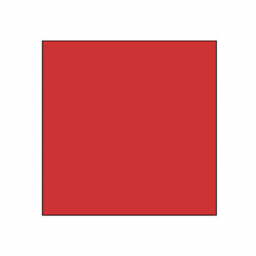# Circumference 72414

Calculate the diagonal and the area of a square if its circumference is 10 centimeters.

u =  3.5355 cm
S =  6.25 cm2

### Step-by-step explanation:Did you find an error or inaccuracy? Feel free to write us. Thank you!

Tips for related online calculators
The Pythagorean theorem is the base for the right triangle calculator.

#### You need to know the following knowledge to solve this word math problem:

We encourage you to watch this tutorial video on this math problem: